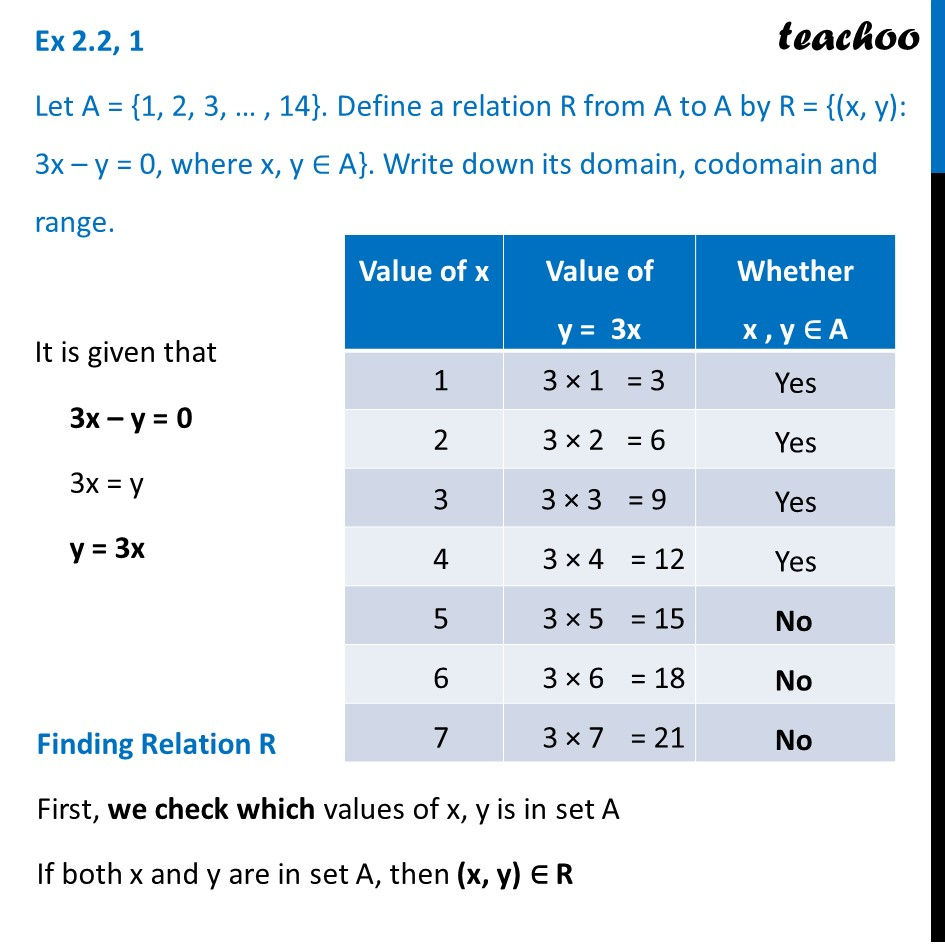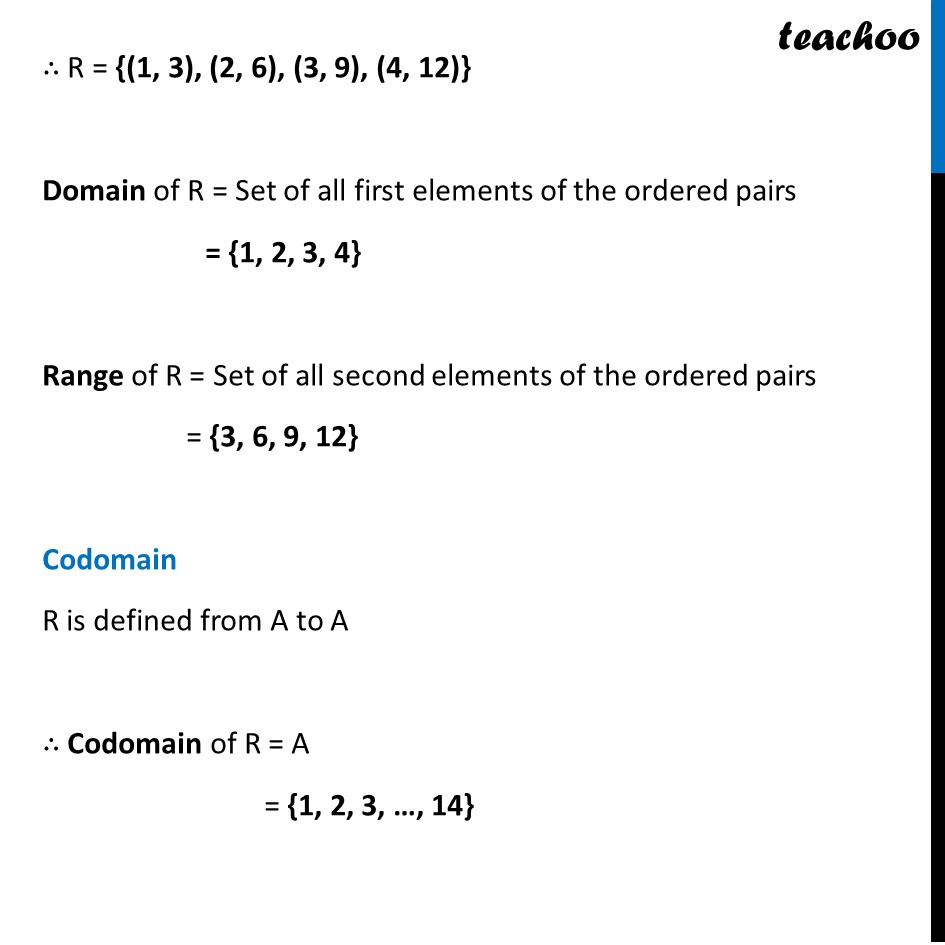Finding Relation - Set-builder form given

Chapter 2 Class 11 Relations and Functions
Concept wiseLearn in your speed, with individual attention - Teachoo Maths 1-on-1 Class

### Transcript

Ex 2.2, 1 Let A = {1, 2, 3, … , 14}. Define a relation R from A to A by R = {(x, y): 3x – y = 0, where x, y ∈ A}. Write down its domain, codomain and range. It is given that 3x – y = 0 3x = y y = 3x Finding Relation R First, we check which values of x, y is in set A If both x and y are in set A, then (x, y) ∈ R ∴ R = {(1, 3), (2, 6), (3, 9), (4, 12)} Domain of R = Set of all first elements of the ordered pairs = {1, 2, 3, 4} Range of R = Set of all second elements of the ordered pairs = {3, 6, 9, 12} Codomain R is defined from A to A ∴ Codomain of R = A = {1, 2, 3, …, 14}